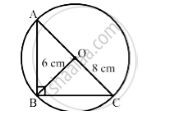# Find the Area of a Right – Angled Triangle, the Radius of Whose, Circumference Measures 8 Cm and the Altitude Drawn to the Hypotenuse Measures 6 Cm. - Mathematics

Find the area of a right – angled triangle, the radius of whose, circumference measures 8 cm and the altitude drawn to the hypotenuse measures 6 cm.

#### SolutionHeight = 6 cm

Area = ?

In a right angled triangle, the center of the circumference is the midpoint of the hypotenuse.

Hypotenuse 2xx(radius of circumference) for a right triangle

=2xx8

=16cm

So, hypotenuse = 16 cm

Now, base = 16 cm and height = 6 cm

Area of the triangle=1/2xxbasexxheight

=1/2xx16xx6

=48 cm^2

Concept: Circumference of a Circle
Is there an error in this question or solution?

#### APPEARS IN

RS Aggarwal Secondary School Class 10 Maths
Chapter 17 Perimeter and Areas of Plane Figures
Q 15